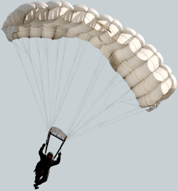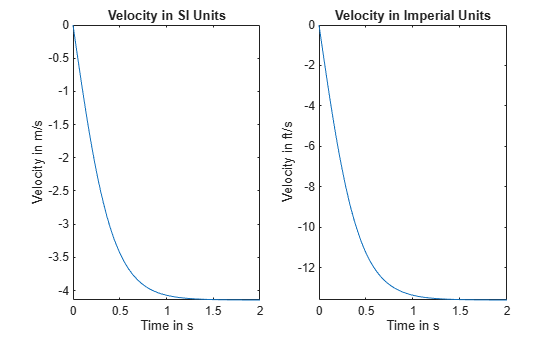Documentation

### This is machine translation

Mouseover text to see original. Click the button below to return to the English version of the page.

## Using Units in Physics

Use units to perform physics calculations in both SI and Imperial units. Compute with units the terminal velocity of a falling paratrooper by modeling the deceleration of velocity due to drag.

### Introduction

Imagine that a paratrooper is dropped from an airplane. Assume there are only two forces acting on the paratrooper, the gravitational force and an opposing drag force from the parachute.The net force acting on the paratrooper can be expressed as :

$\mathrm{mass}\cdot \mathrm{acceleration}=\mathrm{drag}\text{\hspace{0.17em}}\mathrm{force}-\mathrm{gravitational}\text{\hspace{0.17em}}\mathrm{force}$,

$\mathit{m}\frac{\partial }{\partial \mathit{t}}\mathit{v}\left(\mathit{t}\right)={\mathit{c}}_{\mathit{d}}{\mathit{v}\left(\mathit{t}\right)}^{2}-\mathit{m}\text{\hspace{0.17em}}\mathit{g}$,

where

• $m$ is the paratrooper's mass

• g is the acceleration due to gravity

• $v\left(t\right)$is the paratrooper's velocity

• ${c}_{d}$ is the drag constant

Define and Solve the Differential Equation

Define the differential equation describing the balance of forces.

```syms g m c_d syms v(t) eq = m*diff(v(t),t) + m*g == c_d*v(t)^2```
```eq =  ```

Assume that the parachute opens immediately at $\mathit{t}=0$ so that the equation `eq` is valid for all values of $\mathit{t}\ge 0$. Solve the differential equation analytically using `dsolve` with the initial condition $\mathit{v}\left(0\right)=0$.

`velocity = simplify(dsolve(eq, v(0) == 0))`
```velocity =  $-\frac{\sqrt{g} \sqrt{m} \mathrm{tanh}\left(\frac{\sqrt{{c}_{d}} \sqrt{g} t}{\sqrt{m}}\right)}{\sqrt{{c}_{d}}}$```

### Find Units of Drag Constant

Find the units of the drag constant ${c}_{d}$ in SI units. The units of Force are Newton $\left(\mathit{N}\right)$ or expressed in SI base units are $\left(\frac{\mathrm{kg}\cdot \mathit{m}}{{\mathit{s}}^{2}}\right)$. Since they are equivalent they have a unit conversion factor of 1.

```u = symunit; unitConversionFactor(u.N, u.kg*u.m/u.s^2)```
`ans = $1$`

Since the drag force ${\mathit{c}}_{\mathit{d}}{\mathit{v}\left(\mathit{t}\right)}^{2}$ must have the same physical dimension in Newton $\left(\mathit{N}\right)$as the gravitational force $m\phantom{\rule{0.16666666666666666em}{0ex}}g$, the physical dimension of ${c}_{d}$ can be solved for.

```syms drag_units_SI drag_units_SI = simplify(solve(drag_units_SI * (u.m / u.s)^2 == u.N))```
```drag_units_SI =  $1 \frac{\mathrm{kg}\mathrm{"kilogram - a physical unit of mass."}}{\mathrm{m}\mathrm{"meter - a physical unit of length."}}$```

### Estimate the terminal velocity

Assume:

• Paratrooper's mass $\mathit{m}\text{\hspace{0.17em}}=\text{\hspace{0.17em}}70\text{\hspace{0.17em}}\mathrm{kg}$

• Acceleration due to gravity $\mathit{g}\text{\hspace{0.17em}}=\text{\hspace{0.17em}}9.81\text{\hspace{0.17em}}\mathit{m}/{\mathit{s}}^{2}$

• Drag coefficient ${\mathit{c}}_{\mathit{d}}\text{\hspace{0.17em}}=\text{\hspace{0.17em}}40\text{\hspace{0.17em}}\mathrm{kg}/\mathit{m}$

Substitute these values into the velocity equation and simplify the result.

`vel_SI = subs(velocity,[g,m,c_d],[9.81*u.m/u.s^2, 70*u.kg, 40*drag_units_SI])`
```vel_SI =  $-\frac{\mathrm{tanh}\left(\frac{t \sqrt{40 \frac{\mathrm{kg}\mathrm{"kilogram - a physical unit of mass."}}{\mathrm{m}\mathrm{"meter - a physical unit of length."}}} \sqrt{\frac{981}{100} \frac{\mathrm{m}\mathrm{"meter - a physical unit of length."}}{{\mathrm{s}\mathrm{"second - a physical unit of time."}}^{2}}}}{\sqrt{70 \mathrm{kg}\mathrm{"kilogram - a physical unit of mass."}}}\right) \sqrt{70 \mathrm{kg}\mathrm{"kilogram - a physical unit of mass."}} \sqrt{\frac{981}{100} \frac{\mathrm{m}\mathrm{"meter - a physical unit of length."}}{{\mathrm{s}\mathrm{"second - a physical unit of time."}}^{2}}}}{\sqrt{40 \frac{\mathrm{kg}\mathrm{"kilogram - a physical unit of mass."}}{\mathrm{m}\mathrm{"meter - a physical unit of length."}}}}$```
`vel_SI = simplify(vel_SI)`
```vel_SI =  $-\frac{3 \sqrt{763} \mathrm{tanh}\left(\frac{3 \sqrt{763} t}{35} \frac{1}{\mathrm{s}\mathrm{"second - a physical unit of time."}}\right)}{20} \frac{\mathrm{m}\mathrm{"meter - a physical unit of length."}}{\mathrm{s}\mathrm{"second - a physical unit of time."}}$```

Compute a numerical approximation to 3 significant digits.

```digits(3) vel_SI = vpa(vel_SI)```
```vel_SI =  $-4.14 \mathrm{tanh}\left(2.37 t \frac{1}{\mathrm{s}\mathrm{"second - a physical unit of time."}}\right) \frac{\mathrm{m}\mathrm{"meter - a physical unit of length."}}{\mathrm{s}\mathrm{"second - a physical unit of time."}}$```

The paratrooper soon approaches a constant velocity when the gravitational force is balanced by the drag force. This is called terminal velocity and occurs when the drag force from the parachute is roughly equivalent to the gravitational force, and there is no further acceleration. Find the terminal velocity by using `limit` as $\mathit{t}⟶\infty$.

`vel_term_SI = limit(vel_SI, t, Inf)`
```vel_term_SI =  $-4.14 \frac{\mathrm{m}\mathrm{"meter - a physical unit of length."}}{\mathrm{s}\mathrm{"second - a physical unit of time."}}$```

Rewrite Velocity Using Imperial units

Finally, we rewrite the velocity function from SI units to Imperial units.

`vel_Imperial = rewrite(vel_SI,u.ft)`
```vel_Imperial =  $-13.6 \mathrm{tanh}\left(2.37 t \frac{1}{\mathrm{s}\mathrm{"second - a physical unit of time."}}\right) \frac{\mathrm{ft}\mathrm{"foot - a physical unit of length."}}{\mathrm{s}\mathrm{"second - a physical unit of time."}}$```

Rewrite the terminal velocity.

`vel_term_Imperial = rewrite(vel_term_SI,u.ft)`
```vel_term_Imperial =  $-13.6 \frac{\mathrm{ft}\mathrm{"foot - a physical unit of length."}}{\mathrm{s}\mathrm{"second - a physical unit of time."}}$```

### Plot Velocity Over Time

To plot deceleration, we measure the time t in seconds and replace t by t = T s, where T is a dimensionless symbolic variable.

```syms T vel_SI = subs(vel_SI, t, T*u.s)```
```vel_SI =  $-4.14 \mathrm{tanh}\left(2.37 T\right) \frac{\mathrm{m}\mathrm{"meter - a physical unit of length."}}{\mathrm{s}\mathrm{"second - a physical unit of time."}}$```
`vel_Imperial = rewrite(vel_SI, u.ft)`
```vel_Imperial =  $-13.6 \mathrm{tanh}\left(2.37 T\right) \frac{\mathrm{ft}\mathrm{"foot - a physical unit of length."}}{\mathrm{s}\mathrm{"second - a physical unit of time."}}$```

Separate the expression from the units by using `separateUnits`. Plot the expression using `fplot`. Convert the units to strings for use as plot labels using `symunit2str` .

```[data_SI, units_SI] = separateUnits(vel_SI); [data_Imperial, units_Imperial] = separateUnits(vel_Imperial);```

We see that the paratrooper's velocity approaches its steady state when $\mathit{t}>1$. Show how the velocity approaches terminal velocity by plotting the velocity over the range $0\text{\hspace{0.17em}}\le \mathit{T}\text{\hspace{0.17em}}\le 2$.

```subplot(1,2,1) fplot(data_SI,[0 2]) title('Deceleration in SI Units') xlabel('Time in s') ylabel(['Velocity in ' symunit2str(units_SI)]) subplot(1,2,2) fplot(data_Imperial,[0 2]) title('Deceleration in Imperial Units') xlabel('Time in s') ylabel(['Velocity in ' symunit2str(units_Imperial)])```